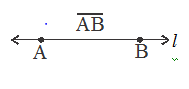# Some Basic Elements Of Geometry

## Basics of Geometry of Class 6

### Some Basic elements of Geometry

There are three basic elements which form the building blocks of Geometry (i) Point  (ii) Line (iii) Plane

Point

A point has position and its location can be ascertained. If has no length, breadth or thickness. A dot made with a sharp pencil may be taken as roughly representing a point.

Some examples: The corners of a square, the tip of an ice cone etc, are examples of a point.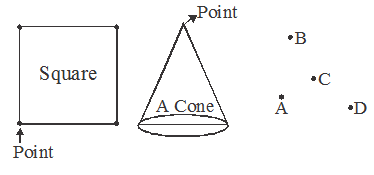Points are denoted by capital letters like A, B, C, D etc. and read as ‘Point A’, ‘Point B’, ‘Point C’, ‘Point D’.

### Line

The basic concept of a line is its straightness and it extends indefinitely in both directions.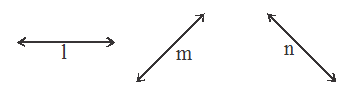Collinear Points

Points lying on the same line are called collinear points.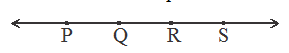### Plane

A plane is a flat surface extending in definitely in all directions.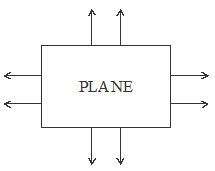### Intersecting and parallel planes

We know that the two adjacent walls of a room intersect along a line, while the floor and ceiling of a room do not intersect.

The planes which do not intersect are called parallel planes.

The planes which intersect along a line are called intersecting planes

### CO-Planar Lines

Two or more lines which lie in the same plane are called coplanar lines.

Example: In plane ABC, lines l, m, n, is there. So, l, m, n are called coplanar lines.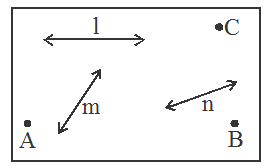### Concurrent Lines

Three or more lines passing through the same point are called con-current lines. The common point is called the point of concurrence.

Examples:

l, m, n and p are con-current lines passing through the common point p.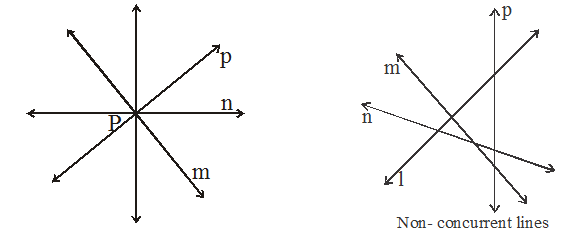### Line Segment

A line segment is a part of a line. It has two end points and a definite length.

Let A and B be two points in a plane and L be the line passing through A and B. The part of the line l between points A an B is called the line segment AB. It is denoted by AB or line segment AB.